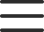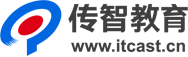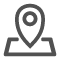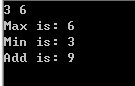# C/C++函数指针简单讲解

1) 函数指针的定义形式中的数据类型是指函数的返回值的类型。

2) 区分下面两个语句：

int (*p)(int a, int b); //p是一个指向函数的指针变量，所指函数的返回值类型为整型

int *p(int a, int b); //p是函数名，此函数的返回值类型为整型指针

3) 指向函数的指针变量不是固定指向哪一个函数的，而只是表示定义了一个这样类型的变量，它是专门用来存放函数的入口地址的;在程序中把哪一个函数的地址赋给它，它就指向哪一个函数。

4) 在给函数指针变量赋值时，只需给出函数名，而不必给出参数。

5) 在一个程序中，指针变量p可以先后指向不同的函数，但一个函数不能赋给一个不一致的函数指针(即不能让一个函数指针指向与其类型不一致的函数)。

p1 = fn1; //正确

p2 = fn2; //正确

p1 = fn2; //产生编译错误

6) 定义了一个函数指针并让它指向了一个函数后，对函数的调用可以通过函数名调用，也可以通过函数指针调用(即用指向函数的指针变量调用)。

7) 函数指针只能指向函数的入口处，而不可能指向函数中间的某一条指令。不能用*(p+1)来表示函数的下一条指令。

8) 函数指针变量常用的用途之一是把指针作为参数传递到其他函数。

#include

using namespace std;

int max(int x, int y); //求最大数

int min(int x, int y); //求最小数

int add(int x, int y); //求和

void process(int i, int j, int (*p)(int a, int b)); //应用函数指针

int main()

{

int x, y;

cin>>x>>y;

cout<<"Max is: ";

process(x, y, max);

cout<<"Min is: ";

process(x, y, min);

cout<<"Add is: ";

process(x, y, add);

return 0;

}

int max(int x, int y)

{

return x > y ? x : y;

}

int min(int x, int y)

{

return x > y ? y : x;

}

int add(int x, int y)

{

return x + y;

}

void process(int i, int j, int (*p)(int a, int b))

{

cout<

}